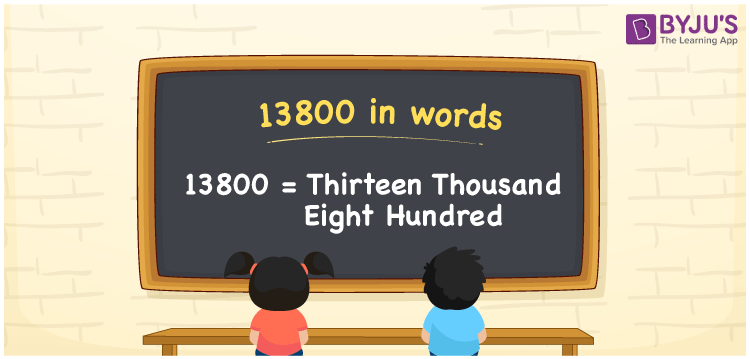# 13800 in Words

13800 in words is written as Thirteen thousand eight hundred. In both the International System of Numerals and the Indian System of Numerals, 13800 in words is written as Thirteen thousand eight hundred. The number 13800 is a Cardinal Number as it represents some quantity. For example, “he has 13800 rupees in his bank account”.

 13800 in words Thirteen thousand eight hundred Thirteen thousand eight hundred in numerical form 13800

## 13800 in English Words

13800 in English words is read as “Thirteen thousand eight hundred.”## How to Write 13800 in Words?

To write 13800 in words, we shall use the place value chart. In the place value chart, put 1 in the ten thousands, 3 in the thousands, 8 in the hundreds, and 0 in the tens and the ones, respectively. Let us make a place value chart to write the number 13800 in words.

 Ten Thousands Thousands Hundreds Tens Ones 1 3 8 0 0

Thus, we can write the expanded form as

1 × Ten Thousand + 3 × Thousand + 8 × Hundred + 0 × Ten + 0 × One

= 1 × 10000 + 3 × 1000 + 8 × 100 + 0 × 10 + 0 × 1

= 10000 + 3000 + 800 + 0 + 0

= 13800

= Thirteen thousand eight hundred.

13800 is the natural number that is succeeded by 13799 and preceded by 13801.

13800 in words – Thirteen thousand eight hundred

• Is 13800 an odd number? – No
• Is 13800 an even number? – Yes
• Is 13800 a perfect square number? – No
• Is 13800 a perfect cube number? – No
• Is 13800 a prime number? – No
• Is 13800 a composite number? – Yes

## Frequently Asked Questions on 13800 in Words

Q1

### How to write 13800 in words?

13800 in words is written as Thirteen thousand eight hundred.
Q2

### How to write 13800 in words in the International and Indian System of Numerals?

In both, the system of numerals, 13800 in words, is written as Thirteen thousand eight hundred.
Q3

### How to write 13800 in a place value chart?

In the place value chart, write 1 in the ten thousands, 3 in the thousands, 8 in the hundreds, and 0 in the tens and the ones, respectively.
Test your Knowledge on 13800 in Words